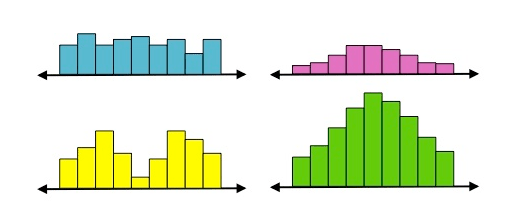PURCHASE A DIGITAL COPY PURCHASE A HARD COPY Lesson 1 Introduction to Statistical Research Methods Lesson 2 Visualizing Data Lesson 3 Central Tendency Lesson 4 Variability Lesson 5 Standardizing Lesson 6 Normal Distribution Lesson 7 Sampling Distributions Lesson 8 Estimation Lesson 9 Hypothesis Testing Lesson 10 t-Tests for Dependent Samples Lesson 11 t-Tests for Independent Samples Lesson 12 Intro to One-Way ANOVA Lesson 13 One-Way ANOVA: Test significance of differences Lesson 14 Correlation Lesson 15 Linear Regression Lesson 16 Chi-Squared Tests Afterward Index

Let’s say you have these two salary distributions. They have the same mean, median, and mode.The main difference between them is the spread. One way we can see this is by looking at the range of each dataset.

The range in the top distribution is \$78,600 – \$21,180 = \$57,420
The range in the bottom distribution is \$116,020 – \$7350 = \$108,670

You can see from the range that the salaries of the general population are much more dispersed whereas salaries of those with social networking accounts are more concentrated.

While range is one method to describe the spread of data, it has limitations. Namely, if the data includes more people within that range, the range will not change. Take these four distributions, for example.They all have the exact same range, and similar means, medians, and modes, but they all have very different shapes. The top left is relatively uniform; the top right is normal; the bottom left is bimodal; and the bottom right is also normal, but with more data points. Therefore, you see that the range itself does not adequately describe the spread of data.

To continue learning, purchase Street-Smart Stats: A Friendly Introduction to Statistical Research Methods.